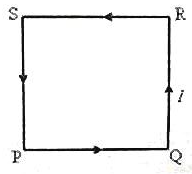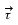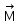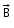Courses

# Test: Torque On Current Carrying Loop

## 10 Questions MCQ Test Physics Class 12 | Test: Torque On Current Carrying Loop

Description
This mock test of Test: Torque On Current Carrying Loop for JEE helps you for every JEE entrance exam. This contains 10 Multiple Choice Questions for JEE Test: Torque On Current Carrying Loop (mcq) to study with solutions a complete question bank. The solved questions answers in this Test: Torque On Current Carrying Loop quiz give you a good mix of easy questions and tough questions. JEE students definitely take this Test: Torque On Current Carrying Loop exercise for a better result in the exam. You can find other Test: Torque On Current Carrying Loop extra questions, long questions & short questions for JEE on EduRev as well by searching above.
QUESTION: 1

### A device which converts electrical energy into mechanical energy is called:​

Solution:

A device which converts electrical energy into mechanical energy is called an electrical motor.
The working principle of an electric motor depends on the magnetic and electric field interaction.
There are two types of electric motor and they are:
AC motor: Converts alternating current into mechanical power. Linear motor, synchronous motor, and induction motor are the three types of AC motor.
DC motor: Converts direct current into mechanical power. Self-excited motor and separately excited are the two classifications of DC motor.

QUESTION: 2

### A conductor of length l, carrying current I and placed in a magnetic field B experiences a force F given by

Solution:

current (i) in a magnetic field ( Bexperiences a force ( Fgiven by the equation

F = i (l × B)

or F = ilB sin θ,

where l is the length of the wire, represented by a vector pointing in the direction of the current.

QUESTION: 3

### A wire 40 cm long carries a current of 20 A. It is bent into a loop and placed with its plane perpendicular to a magnetic field having a flux density of 0.52 T. What is the torque on the loop if it is bent into a square.​

Solution:

a2, = I A B
The 40cm of wire can bent into a square with slides of 10cm
A=(10cm)2=100 cm2
τ=I A B
τ=(20A)(100cm2)(0.52T)
τ=1040 A cm2T[1m2/104 cm2]
τ=0.1040 N-m

QUESTION: 4

A charge moves through a magnetic field with a certain velocity. Direction of force can be obtained by applying :

Solution:

Answer :- c

Solution :- When a charged particle moves in a magnetic field, a force is exerted on the moving charged particle. The formula for the force depends on the charge of the particle, and the cross product of the particle's velocity and the magnetic field.

QUESTION: 5

A plane square loop PQRS of side ‘a’ made of thin copper wire has ‘n’ turns and it carries a direct current ‘I’ ampere in the direction shown in the adjoining figure. This wire loop is placed in a magnetic field of flux density ‘B’ tesla, which is directed perpendicularly in to the plane of the loop. What is the torque acting on the loop?Solution:

The magnetic moment of this loop is in direction perpendicular to the plane of loop and coming out of the plane. The magnetic field is also directed perpendicularly into the loop. So, magnetic moment magnetic field are antiparallel. The torque acting on the loop, which is given by the cross product of moment and field, is zero since sin 180 = 0

QUESTION: 6

Torque on a current loop is by= n(x). With ishere?

Solution:
QUESTION: 7

A wire of length l, carrying current is bent into a loop and placed with its plane perpendicular to a magnetic field. In which of the following shapes, is the torque acting on the loop maximum?

Solution:

The torque on a current loop depends upon the area of the current loop, when the magnetic field is perpendicular to the plane of the loops the torque has its maximum value,
Τ=I A B
We know I and B for all these cases but A depends upon the geometry. The circle has the greatest area so it should provide the greatest torque.

QUESTION: 8

A current loop of area 0.01m2 and carrying a current of 100 A is held parallel to a magnetic field of intensity 0.1 tesla. The torque in Nm acting on the loop is:

Solution:

A=0.01m2, I=100A, B=0.1T, θ=90o,sinθ=1
Now magnetic moment M =I×A
Torque, τ=M×B
=MBsinθ
=IAB
=100×0.01×0.1=0.1Nm.

QUESTION: 9

A coil in shape of an equilateral triangle if side ‘|’ is suspended between the pole pieces of a permanent magnet such that the magnetic field B is in the plane of the coil. If due to a current I in the triangle, a torque Syntax error from line 1 column 49 to line 1 column 73. Unexpected ‘mathsize’. acts on it, the side of the triangle is:

Solution:

Correct Answer :- a

Explanation : The current flowing clockwise in an equilateral triangle has a magnetic field in the direction of k^.

τ = BiNAsinθ

τ = BiNAsin90o

τ = Bi × (√3)/4I2 × 1

[∵ Area of equilateral triangle = (√3)/4I2 and N = 1]

(assuming)

⇒ I2 = 4τ/(3Bi)

=> I = 2[τ/Bi√3]1/2

QUESTION: 10

A charge of +10Âµ coulomb enters a uniform magnetic field parallel to its direction. What will happen to the charge?​

Solution:

As the magnetic field is parallel to the charge, no magnetic Lorentz force will act on it and it will move undeviated with constant velocity.